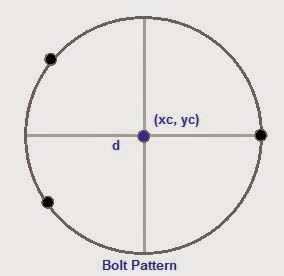Bolt Pattern

## Description

The program BOLTPAT will calculate a bolt pattern given:
X = center, X coordinate
Y = center, Y coordinate
N = number of bolts
D = diameter of the circle

No angle of rotation is assumed.

The OC-Distance between each bolt is calculated by calculating
the linear distance between neighboring bolts.

Output:
Each point where the bolt should be placed. In addition, the on-
center distance between bolts is displayed. Finally, each of the
coordinates will be presented in a list.

## Screenshots## Archive Contents

Name Size
bolt pattern diagram.jpg 10.1 KB
BOLTPAT.hpprgm 1.1 KB
File Size
10.8 KB

Author
EdPi314
8 years, 4 months ago

Rating
10/10 (1 vote)